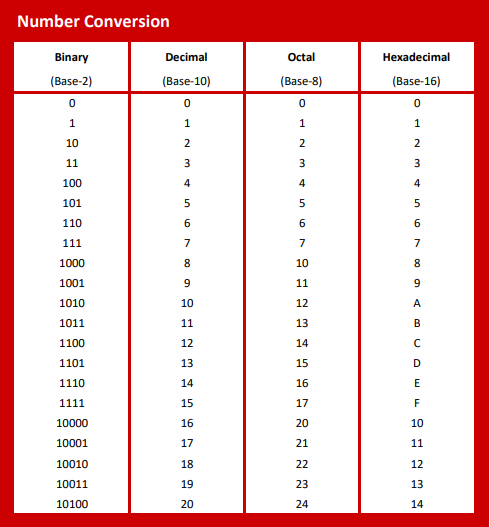# Number System Conversion Calculator

Common programming number systems include Binary (Base 2), Decimal (Base 10), Octal (Base 8), and Hexadecimal (Base 16). The number system conversion calculator allows the user to enter a number in any system and convert it to the others. It also functions as a binary bit shift calculator allowing you to change the values by shifting bits right or left or by changing individual bits.

Decimal
Octal
BinaryBinary Bit Manipulation

31
24
23
16
15
8
7
0
Number Conversion Explained

The decimal numbering system is what we use on a daily basis. In the decimal numbering system the position of the digits represent a power of 10 (base 10). This means that as you move left from the least significant bit, you will increment to the next position after you reach 9. A value of 9 represents 9 “ones”, while 10 represents 1 “ten”.

Binary is a base 2 system in which only 1’s and 0’s are used. Each position represents a step of 1. A binary number of 1 is followed sequentially with 10 (1 in the “2’s” place and “0” in the ones). Next would be 11 (1 in the 2’s, +1 in the ones). 100 would be Decimal 4 (1 in the fours, 0 in the 2, 0 in the ones). The biggest advantage of a binary numbering system when it comes to programming is that it’s very easy for circuits to represent the two states. In electronics, the 1’s and 0’s can be used as off or on states. This makes Binary the base for all programming. Binary’s downfalls come from the fact that binary numbers end up being very long if the number is large.

The Octal system is base 8 which means the positional indication of the numbers (from LSB) go 1’s, 8’s, 64’s, etc. For example – in the octal numbering system 135 breaks down as 1x64 + 3x8 + 5x1 for a total of 93. The octal system is less popular today and has largely been replaced by the base 16 Hexadecimal system.

The Hexadecimal system is based on a base of 16 and uses the numbers 0-9 and the letters A~F. In this system the “ones” position increments 0-9 but “10” is represented by the letter A, 11 by B, etc. The biggest advantage of the hex system is that it is an easier way to represent very large numbers. A hex value of 4B6 breaks down to 4 (binary 0100) B (binary 1011) 6 (binary 0110). In this way it can take a very long binary string and condense it into an easier to read format.

×